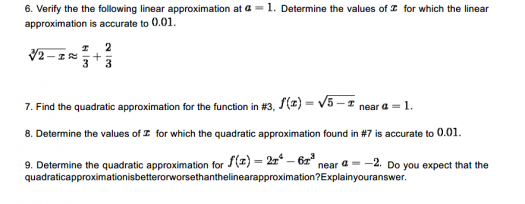# Calculus

## A Beginner's Guide to Differentiation and Integration: Unlocking the Power of Calculus

Introduction:
Welcome to the Beginner's Guide to Differentiation and Integration, where we'll explore the fundamental concepts of calculus that form the backbone of mathematical analysis. Whether you're a student just starting to delve into calculus or someone looking to refresh their understanding, this guide will provide you with a solid foundation in differentiation and integration. So, let's embark on this exciting journey into the world of calculus!

## Differential Equation: $(1-xy)^{-2} dx + \left[ y^2 + x^2 (1-xy)^{-2} \right] dy = 0$

Hello, can anyone solve this equation?

I can't figure it out,

$(1-xy)^{-2} dx + \left[ y^2 + x^2 (1-xy)^{-2} \right] dy = 0$

Thanks.

## Differential Equation: $y' = x^3 - 2xy$, where $y(1)=1$ and $y' = 2(2x-y)$ that passes through (0,1)

Can anyone solve this D. E.?

y' = x^3 - 2xy, where y(1)=1

and

y' = 2(2x-y) that passes through (0,1)

## Basic Cal.

Hi! I need help with these.## Help a Calculus dummy.

https://ibb.co/g9Vk3b

https://ibb.co/fZh4ww

How do I solve these? Help po.

## Basic Calculus

Help me find the limits of these:

https://imgur.com/a/yobgF

Help me identify all asymptotes and the end behavior of the graph:

https://imgur.com/a/JxJF2

## Families of Curves: family of circles with center on the line y= -x and passing through the origin

Find the diff equation of family of circles with center on the line y= -x and passing through the origin.

THANK YOU :)

## Applications Of Differential Equations

The radial stress p at distance r for the axis of the thick cylinder subjected to internal pressure is given by p + r (dp/dr) = A − p where A is a constant. If p = p0 at the inner wall r = r1 and is negligible (p = 0) at the outer wall r = r2 find an expression for p.

## Eliminating arbitrary constants

kindly help me with my homework. eliminate the following arbitrary constants...
y= x^2 + c1 e^3x + c2 e^3x.